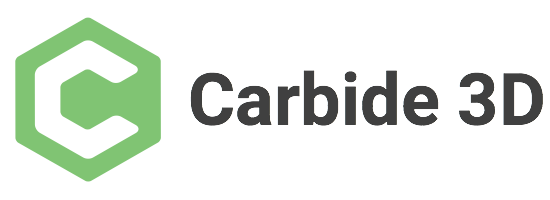How to access the Boolean function?

So in the basic carbide create guide it looks as if the boolean option is just supposed to show up when you make a shape, or two shapes. However I cannot find any option for booleans. Can someone let me know what I am doing wrong here?

I am trying to round out the edges on some .4" thick rectangles.

Select two overlapping shapes

so I guess that is one of the issues, I cannot select two shapes at once apparently… normally this would be done by holding shift while selecting (in other 3D programs at least).

I have attached an image of the round out I am trying to achieve.

Hold the control key while selecting the second shape.

You want to draw that differently — use two rectangles creating a notch, then draw the rounding circle, then union.

Alternately, draw a square at the corner, then the circle, then subtract the circle from the square, then subtract the notched square from the rectangle.

1 Like

ok so if i understand, make a square overlapping the exact corner, then a circle inside that square? Sorry very new to this so stick with me.

As for the rectangles, how should they be overlapped? Images would be amazing if it’s not to much to ask.

Either way thank you so much.

Using a square: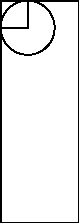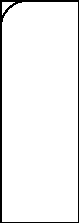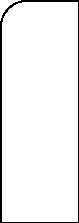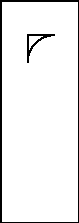1 Like

Two overlapping rectangles and a circle and unioning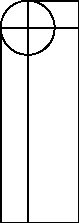1 Like

AHHHH, ok now it makes complete sense. Thank you so much, I owe you a brew or two :: )